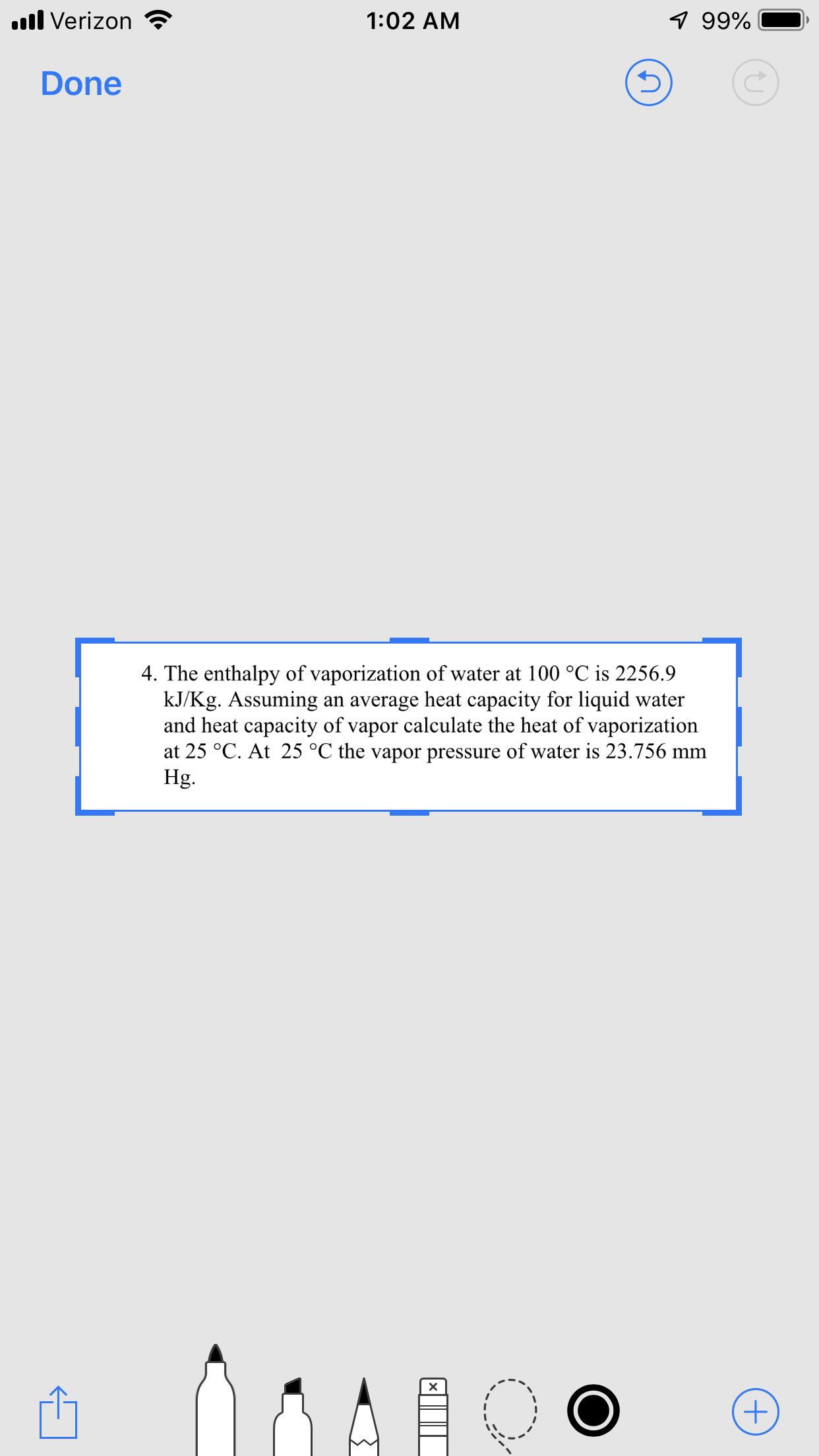# 1:02 AMll Verizon99%-Done4. The enthalpy of vaporization of water at 100 °C is 2256.9kJ/Kg. Assuming an average heat capacity for liquid waterand heat capacity of vapor calculate the heat of vaporizationat 25 °C. At 25 °C the vapor pressure of water is 23.756 mmHg.

Question
9 viewshelp_outlineImage Transcriptionclose1:02 AM ll Verizon 99%- Done 4. The enthalpy of vaporization of water at 100 °C is 2256.9 kJ/Kg. Assuming an average heat capacity for liquid water and heat capacity of vapor calculate the heat of vaporization at 25 °C. At 25 °C the vapor pressure of water is 23.756 mm Hg. fullscreen
check_circle

star
star
star
star
star
1 Rating
Step 1

Watson equation is to be used as written below. Here,

ΔH1 is enthalpy of vaporization at T1.

ΔH2 is enthalpy of vaporization at T2.

Tr is reduced temperature given by the relation, T/Tc, where Tc is the critical temperature given in physical property data tables.

Step 2

Critical temperature for water is taken as: Tc = 647.1 K. Calculate reduced temperature at both T1 and T2 as:

Step 3

Value of ΔH1 is given as 2256.9 kJ/kg. ΔH2 is ...

### Want to see the full answer?

See Solution

#### Want to see this answer and more?

Solutions are written by subject experts who are available 24/7. Questions are typically answered within 1 hour.*

See Solution
*Response times may vary by subject and question.
Tagged in

### Chemical Engineering